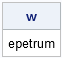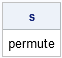My previous post on creating a random permutation started me thinking about word games. My wife loves to solve the daily Jumble® puzzle that runs in our local paper. The puzzle displays a string of letters like MLYBOS, and you attempt to unscramble the letters to make an ordinary word.

You can scramble a word by permuting the letters. Similarly, you can think of unscrambling the letters as the process of finding a permutation that reveals a word.

My previous post included a SAS/IML module that generates a random permutation. The relevant code is reproduced below:

```proc iml; /** generate one random permutation with N>0 elements **/ start RandPerm(N); u = 1:N; /** allocate u **/ call randgen(u, "Uniform"); /** fill with random numbers **/ return(rank(u)); /** return rank (order) of elements **/ finish;```

You can use the RandPerm module to randomly permute the letters of a word. [Editor's Note 13AUG2011: In SAS 9.3, you can use the RANPERM function.] The SUBSTR function and the ROWCATC function are useful for creating a module that takes a word and returns a scrambled version of that word:

```/** given a word, randomly scramble the letters **/ start RandScramble( s ); n = nleng(s); r = RandPerm(n); /** create character array with one element per character **/ w = j(1, n, " "); /** allocate character array **/ do i = 1 to n; w[i] = substr(s, r[i], 1); /** permute characters **/ end; return(rowcatc(w)); /** concatenate to a single word **/ finish;   call randseed(123); /** optional: make reproducible **/ w = RandScramble( "permute" ); print w;```You can use the same idea (and practically the same code!) to "undo" the permutation. Specifically, the following module takes a (scrambled) word and a permutation, and returns the sequence of letters that result from applying the permutation. If you can guess the correct permutation, the underlying word is revealed!

```/** apply a given permutation to a string of characters **/ start PermuteChars( w, perm ); n = nleng(w); s = j(1, n, " "); /** allocate character array **/ do i = 1 to n; s[i] = substr(w, perm[i], 1); end; return(rowcatc(s)); finish;   p = {2 3 5 7 6 4 1}; s = PermuteChars(w, p); print s;```Because the two modules are so similar, the careful programmer will reexamine the modules, realize that the PermuteChars module is more general, and therefore will rewrite the RandScramble module as follows:

```/** Version 2: given a word, randomly scramble the letters **/ start RandScramble( s ); r = RandPerm(nleng(s)); return (PermuteChars(s, r)); finish;```

This is an example of "green programming": reduce, reuse, and recycle!

And in case you are wondering, the permutation that unscrambles the letters in the first paragraph (MLYBOS) is
{6 3 1 4 5 2}.

ShareDistinguished Researcher in Computational Statistics

Rick Wicklin, PhD, is a distinguished researcher in computational statistics at SAS and is a principal developer of SAS/IML software. His areas of expertise include computational statistics, simulation, statistical graphics, and modern methods in statistical data analysis. Rick is author of the books Statistical Programming with SAS/IML Software and Simulating Data with SAS.

1.2.